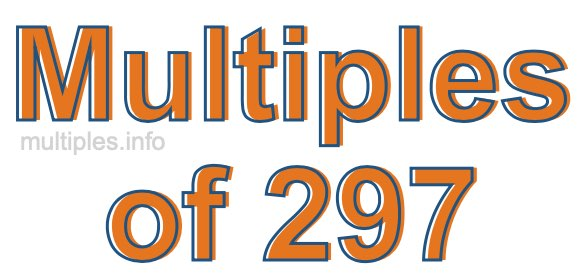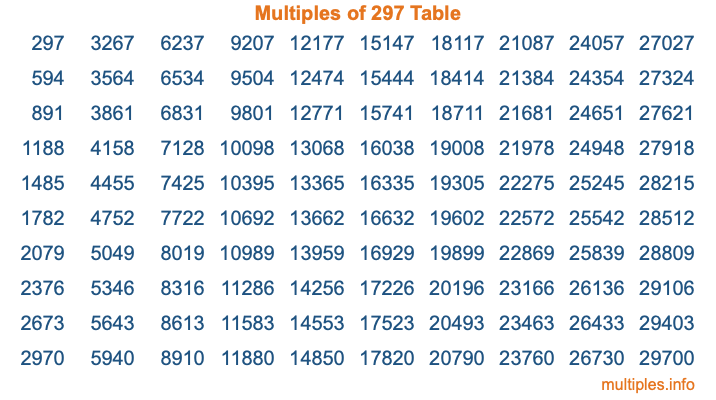Multiples of 297Welcome to the Multiples of 297 page. Here we will first teach you everything you will ever need to know about the multiples of 297, and then give you a study guide summary of everything we taught you to make sure you remember it all. Use this page to look up facts and learn information about the multiples of 297. This page will make you a multiples of two hundred ninety-seven expert!

Definition of Multiples of 297
Multiples of 297 are all the numbers that when divided by 297 equal an integer. Each of the multiples of 297 are called a multiple. A multiple of 297 is created by multiplying 297 by an integer.

Therefore, to create a list of multiples of 297, you start with 1 multiplied by 297, then 2 multiplied by 297, then 3 multiplied by 297, and so on for as long as you want. Thus, the list of the first five multiples of 297 is 297, 594, 891, 1188, and 1485. To see a larger list of multiples of 297, see the printable image of Multiples of 297 further down on this page. We also have a category where you can choose any nth multiple of 297.

Multiples of 297 Checker
The Multiples of 297 Checker below checks to see if any number of your choice is a multiple of 297. In other words, it checks to see if there is any number (integer) that when multiplied by 297 will equal your number. To do that, we divide your number by 297. If the the quotient is an integer, then your number is a multiple of 297.

Is  a multiple of 297?

Least Common Multiple of 297 and ...
A Least Common Multiple (LCM) is the lowest multiple that two or more numbers have in common. This is also called the smallest common multiple or lowest common multiple and is useful to know when you are adding our subtracting fractions. Enter one or more numbers below (297 is already entered) to find the LCM.

Check out our LCM Calculator if you need more details about the Least Common Multiple or if you need the LCM for different numbers for adding and subtraction fractions.

nth Multiple of 297
As we stated above, 297 is the first multiple of 297, 594 is the second multiple of 297, 891 is the third multiple of 297, and so on. Enter a number below to find the nth multiple of 297.

th multiple of 297

Multiples of 297 vs Factors of 297
297 is a multiple of 297 and a factor of 297, but that is where the similarities end. All postive multiples of 297 are 297 or greater than 297. All positive factors of 297 are 297 or less than 297.

Below is the beginning list of multiples of 297 and the factors of 297 so you can compare:

Multiples of 297: 297, 594, 891, 1188, 1485, etc.

Factors of 297: 1, 3, 9, 11, 27, 33, 99, 297

As you can see, the multiples of 297 are all the numbers that you can divide by 297 to get a whole number. The factors of 297, on the other hand, are all the whole numbers that you can multiply by another whole number to get 297.

It's also interesting to note that if a number (x) is a factor of 297, then 297 will also be a multiple of that number (x).

Multiples of 297 vs Divisors of 297
The divisors of 297 are all the integers that 297 can be divided by evenly. Below is a list of the divisors of 297.

Divisors of 297: 1, 3, 9, 11, 27, 33, 99, 297

The interesting thing to note here is that if you take any multiple of 297 and divide it by a divisor of 297, you will see that the quotient is an integer.

Multiples of 297 Table
Below is an image of the first 100 multiples of 297 in a table. The table is in chronological order, column by column. The first column has the first ten multiples of 297, the second column has the next ten multiples of 297, and so on.The Multiples of 297 Table is also referred to as the 297 Times Table or Times Table of 297. You are welcome to print out our table for your studies.

Negative Multiples of 297
Although not often discussed or needed in math, it is worth mentioning that you can make a list of negative multiples of 297 by multiplying 297 by -1, then by -2, then by -3, and so on, to get the following list of negative multiples of 297:

-297, -594, -891, -1188, -1485, etc.

Multiples of 297 Summary
Below is a summary of important Multiples of 297 facts that we have discussed on this page. To retain the knowledge on this page, we recommend that you read through the summary and explain to yourself or a study partner why they hold true.

There are an infinite number of multiples of 297.

A multiple of 297 divided by 297 will equal a whole number.

297 divided by a factor of 297 equals a divisor of 297.

The nth multiple of 297 is n times 297.

The largest factor of 297 is equal to the first positive multiple of 297.

297 is a multiple of every factor of 297.

297 is a multiple of 297.

A multiple of 297 divided by a divisor of 297 equals an integer.

297 divided by a divisor of 297 equals a factor of 297.

Any integer times 297 will equal a multiple of 297.

Multiples of a Number
Here you can get the multiples of another number, all with the same attention to detail as we did for multiples of 297 on this page.

Multiples of
Multiples of 298
Did you find our page about multiples of two hundred ninety-seven educational? Do you want more knowledge? Check out the multiples of the next number on our list!